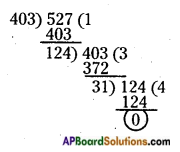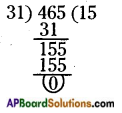AP State Syllabus AP Board 6th Class Maths Solutions Chapter 3 HCF and LCM Ex 3.5 Textbook Questions and Answers.

## AP State Syllabus 6th Class Maths Solutions 3rd Lesson HCF and LCM Ex 3.5

Question 1.
Find the HCF of the following by prime – factorization and by continued division method.
i) 48, 64
ii) 126, 216
iii) 40, 60, 56
iv) 10, 35, 40
i) 48,64
Factorization method:
48 = 2 x 2 x 2 x 2 x 3
64 = 2 x 2 x 2 x 2 x 2 x 2
HCF = 2 x 2 x 2 x 2 = 16
∴ HCF of 48 and 64 is 16.
Continued division method: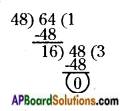HCF = 16ii) 126, 216
Factorization method:
126 = 2 x 3 x 3 x 7
216 = 2 x 2 x 2 x 3 x 3 x 3
HCF = 2 x 3 x 3 = 18
∴ HCF of 126 .and 216 is 18.
Continued division method: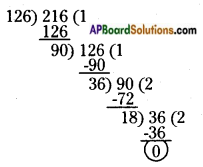HCF = 18

iii) 40, 60, 56
Factorization method:
40 = 2 x 2 x 2 x 5
60 = 2 x 2 x 3 x 5
∴ HCF of 40, 56 and 60 is 4.
Continued division method: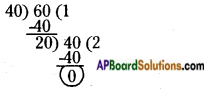First find the HCF of the smallest and the biggest of given numbers i.e., 40 and 60
Now, find the HCF of 20 and the remaining number 56.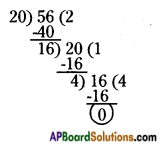HCF = 4iv) 10, 35,40
Factorization method
10 = 2 x 5
35 = 5 x 7 40 = 2 x 2 x 2 x 5
HCF = 5
Continued division method: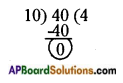First find the HCF of the smallest and biggest numbers of the given numbers, i.e., 10 and 40.
HCF of 10 and 40 is 10.
10 is a factor of 40.
So, HCF (10, 40) is 10.
Now, find HCF of 10 and the remaining number i.e., 10 and 35.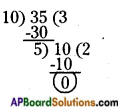HCF of 10 and 35 is 5.
∴ HCF of 10, 35 and 40 is 5.

Question 2.
Two milk cans have 60 and 165 liters of milk. Find a can of maximum capacity which can exactly measure the milk in two cans.
Capacity of first can = 60 l
Capacity of second can = 165 l
Capacity of required can = HCF (60,165)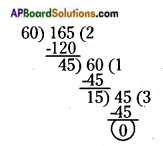HCF of 60 and 165 is 15.
Therefore maximum capacity of the required can is 15 liters.Question 3.
Three different containers contain different quantities of milk whose measures are 403 lit, 465 lit, 527 liters. What biggest measure must be’ there to measure all different quantities in exact number of times?